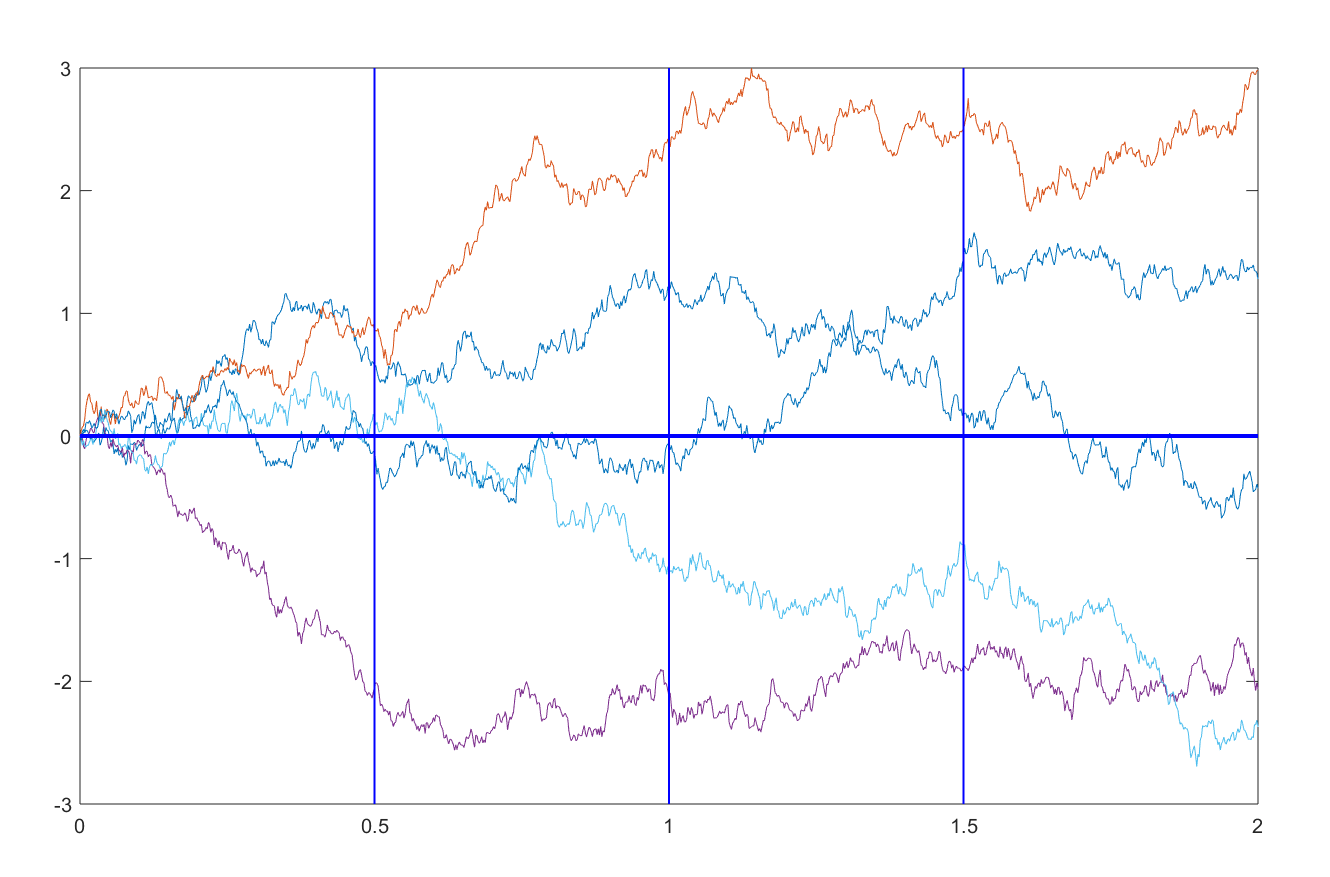13
Oct 18

## Law of iterated expectations: informational aspect

The notion of Brownian motion will help us. Suppose we observe a particle that moves back and forth randomly along a straight line. The particle starts at zero at time zero. The movement can be visualized by plotting on the horizontal axis time and on the vertical axis - the position of the particle.$W(t)$ denotes the random position of the particle at time$t$.Figure 1. Unconditional expectation

In Figure 1, various paths starting at the origin are shown in different colors. The intersections of the paths with vertical lines at times 0.5, 1 and 1.5 show the positions of the particle at these times. The deviations of those positions from$y=0$ to the upside and downside are assumed to be equally likely (more precisely, they are normal variables with mean zero and variance$t$).

### Unconditional expectation

“In the beginning there was nothing, which exploded.” ― Terry Pratchett, Lords and Ladies

If we are at the origin (like the Big Bang), nothing has happened yet and$EW(t)=0$ is the best prediction for any moment$t>0$ we can make (shown by the blue horizontal line in Figure 1). The usual, unconditional expectation$EX$ corresponds to the empty information set.

### Conditional expectationFigure 2. Conditional expectation

In Figure 2, suppose we are at$t=2.$ The dark blue path between$t=0$ and$t=2$ has been realized. We know that the particle has reached the point$W(2)$ at that time. With this knowledge, we see that the paths starting at this point will have the average

(1)$E(W(t)|W(2))=W(2),$$t>2.$

This is because the particle will continue moving randomly, with the up and down moves being equally likely. Prediction (1) is shown by the horizontal light blue line between$t=2$ and$t=4.$ In general, this prediction is better than$EW(t)=0$.

Note that for different realized paths,$W(2)$ takes different values. Therefore$E(W(t)|W(2))$, for fixed$t>2$, is a random variable of$W(2)$. It is a function of the event we condition the expectation on.

### Law of iterated expectationsFigure 3. Law of iterated expectations

Suppose you are at time$t=2$ (see Figure 3). You send many agents to the future$t=3$ to fetch the information about what will happen. They bring you the data on the means$E(W(t)|W(3))$ they see (shown by horizontal lines between$t=3$ and$t=4).$ Since there are many possible future realizations, you have to average the future means. For this, you will use the distributional belief you have at time$t=2.$ The result is$E[E(W(t)|W(3))|W(2)].$ Since the up and down moves are equally likely, your distribution at time$t=2$ is symmetric around$W(2).$ Therefore the above average will be equal to$E(W(t)|W(2)).$ This is the Law of Iterated Expectations, also called the tower property:

(2)$E[E(W(t)|W(3))|W(2)]=E(W(t)|W(2)).$

The knowledge of all of the future predictions$E(W(t)|W(3))$, upon averaging, does not improve or change our current prediction$E(W(t)|W(2))$.

For a full mathematical treatment of conditional expectation see Lecture 10 by Gordan Zitkovic.